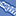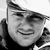# Qlik Compose for Data Lakes

Discussion board for collaboration on Qlik Compose for Data Lakes.

Announcements
QlikWorld 2023, a live, in-person thrill ride. Save \$300 before February 6: REGISTER NOW!
cancel
Showing results for
Did you mean:Contributor

## if with multiple conditions

hey qlikers!

Can we help me whit this?

Created the next field: if the contract is 0 put 3, if it's empty put 3 and if  it's different  put contract  lenght years

if (contract_length_yrs__c= 0, 3,
if (Match(contract_length_yrs__c,'',' '), 3,
if (IsNull(contract_length_yrs__c), 3, contract_length_yrs__c))) as contract_length_yrs__c

now I need to create a field that

if(Outcome='Lost', RFP_est,
if(match (Outcome,'No Bid'),RFP_est,
if(match(Outcome,'RFP Withdrawn by Customer'), RFP_est) ) )

but for some reason when the contract_length_yrs__c is "empty " it doesn't give me "3" and can't calculate  "the RFP_est"

I don't know what I'm doing wrong

Labels (1)
• ### Best Practices

2 RepliesMVP

You can not refer to a created field within the same load statement. Your reference to [contract_length_yrs__c] is the original field value not the value created with your if statement.

You can avoid this problem by using a preceding load

if(Outcome='Lost', RFP_est,

if(match (Outcome,'No Bid'),RFP_est,

if(match(Outcome,'RFP Withdrawn by Customer'), RFP_est) ) ) as FIELDNAME,

*;

if (contract_length_yrs__c= 0, 3,

if (Match(contract_length_yrs__c,'',' '), 3,

if (IsNull(contract_length_yrs__c), 3, contract_length_yrs__c))) as contract_length_yrs__c

FROM Source;Contributor
Author

Hi Vegar,